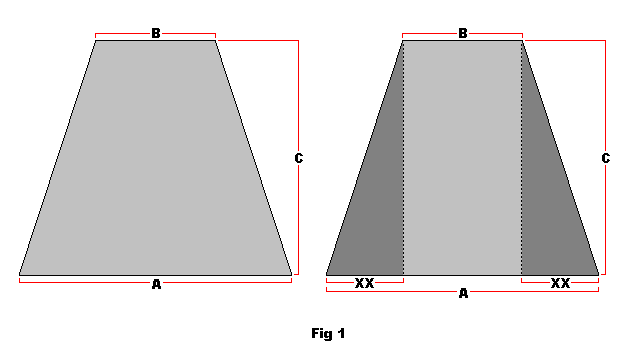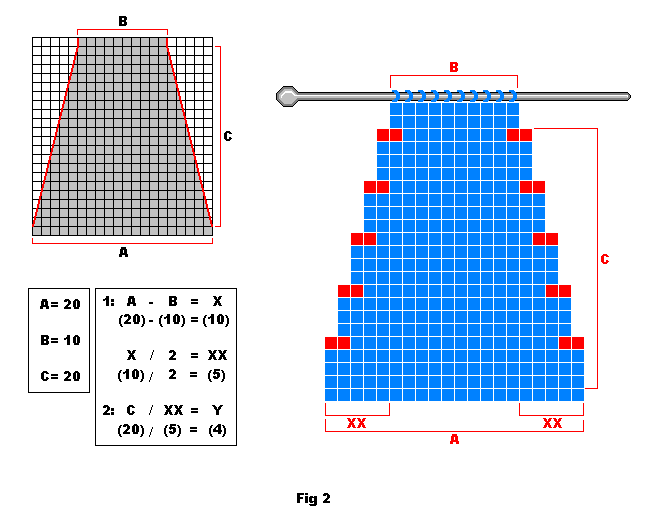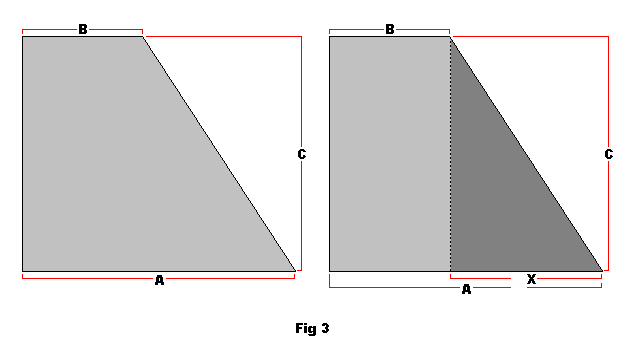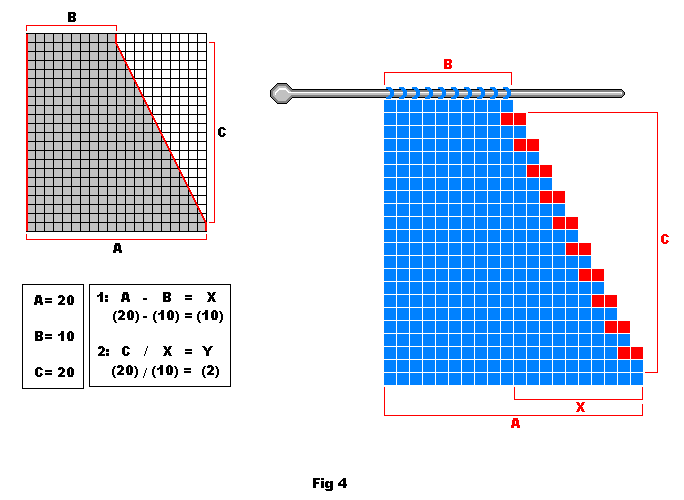# Decreasing width of knitted piece – simple way

Simple decreasing is done by decreasing stitches at the beginning and/or end of the rows. This is done by knitting 2 stitches together, simply reducing 2 stitches to 1. This is the simplest form of shaping.

The measurements you will need are.

A – the amount of stitches you are starting with.

B – The amount of stitched you wish to reduce the knitted piece to (how many you want to end with).

C – The amount of rows you want it to take to reduce the stitches.

For these examples:

A = 20 Stitches

B = 10 Stitches

C = 20 Rows

## How to formulate an even decreasing of stitches on both edges of the knitted piece

To discover how many stitches to decrease over how many rows, you need to use the following formula:

1. (calculation for number of stitches divided by 2 as decrease is done on both edges of the piece)

A – B = X

X / 2 = XX

X is the total amount of stitches we need to reduce by, thus in this example X = 10.

We divide X by 2 because we want to decrease evenly on both edges, thus in this example XX = 5.

2: (calculation for which rows to do the decrease)

C / XX = Y

Y tells us which row we need to be decreasing on, thus in this example Y = 4

Now we know, to achieve the results we desire in this example we need to decrease 1 stitch on each end of the 4th (Y) row for 20 rows.

This example is for a piece knitted in stocking stitch.

Rows 1,2 and 3: Begin with a purl row and knit (stocking stitch) 3 rows (note that the illustrations below start counting one row up).

Row 4: With the front side of the knitted piece facing you knit 2 stitches together (K2 tog). Knit across the row to the last 2 stitches and knit these together (K2 tog). This ends the row which should now be decreased by 2 stitches.

Row 5 and on: Continue this pattern of knitting (decreasing every 4th rows at both ends) until C amount of rows have been completed and B amount of stitches are counted on the needle.

Decreasing is complete. See fig. 1 and 2.A=number of stitches at start, B=number of stitches at end, XX=number of times you need to decrease stitches on both edges of pieceXX=number of times to do a decrease of stitches at both edges, Y=the coefficient for which rows to do the decrease (here 4 as every 4th row should be decreased)

## How to formulate an even decreasing of stitches on one edge of the knitted piece

To discover how many stitches to decrease over how many rows you need to use the following formula:

1: (calculation for number of stitches to decrease)

A – B = X

X is the total amount of stitches we need to reduce by, thus in this example X = 10.

2: (calculation for which rows to do the decrease)

C / X = Y

Y tells us which row we need to be decreasing on, thus in this example Y = 2

Now we know, to achieve the results we desire in this example we need to decrease a stitch at ONE end of every 2nd (Y) row for 20 rows.

If you want the decreasing to be on the right edge of the knit when the front is facing you (as the example shows) then you decrease at the start of the row by knitting the first 2 stitches together.

If you want the decreasing to be on the left edge of the knit when the front is facing you, then you decrease at the end of the row by knitting the last 2 stitches together

This example is for a piece knitted in stocking stitch.

Rows 1: Begin with a purl 1 row (note that the illustrations below start counting one row up).

Row 2: With the front side of the knit facing you knit 2 stitches together (K2 tog) at the beginning OR end of the row depending on which side the decreasing is required to be on.

Row 3 and on: Continue this pattern of knitting until C amount of rows have been completed and B amount of stitches are counted on the needle.

Decreasing is complete. See fig. 3 and 4.A=number of stitches at start, B= number of stitches at end, X= number of stitches to decreaseX= number of stitches to decrease, Y=the coefficient for which rows to do the decrease (here 2 as every 2nd row should be decreased)

This site uses Akismet to reduce spam. Learn how your comment data is processed.